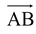# Introduction

## VECTOR ALGEBRA

### Scalars

Quantities having magnitude only but no direction such as mass, length, time etc. are known as scalars.

### Vectors

Quantities having both magnitude and direction such as velocity, acceleration, force etc. are known as vectors represented by a line segmentwith initial point A and terminating point B and its magnitude is represented by.

In the light of this – Equal vectors, co-initial vectors, zero-vector and unit vectors are self-explanatory.